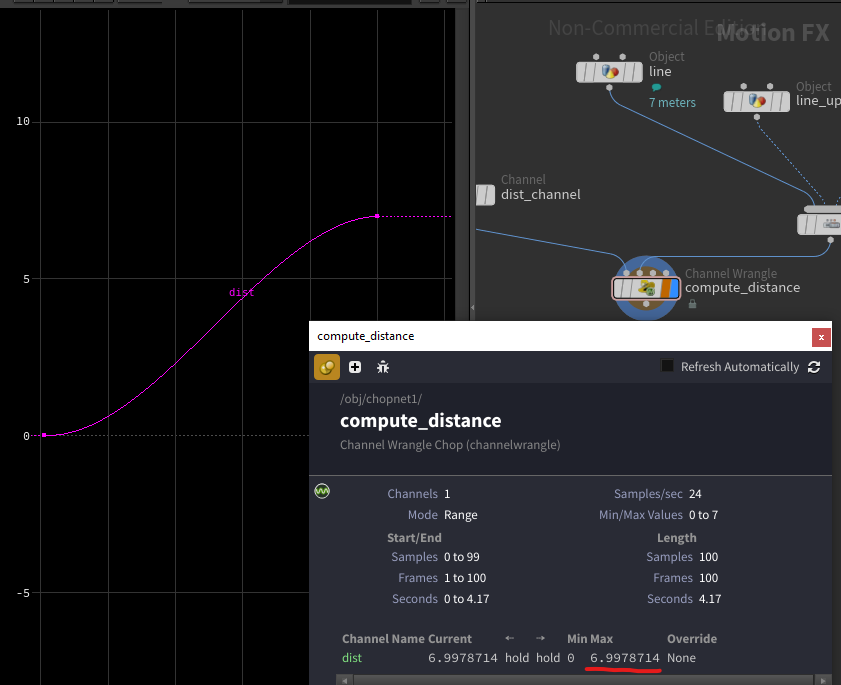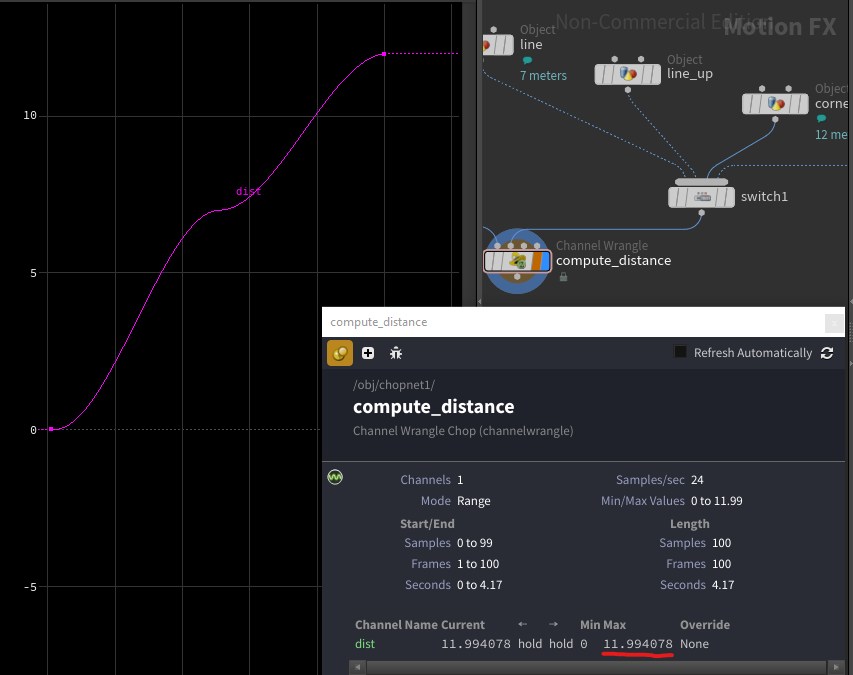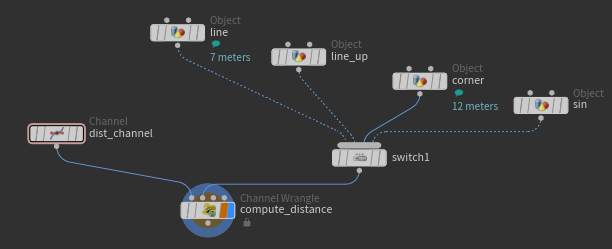## Recommended Posts

Attached hip file, for a sphere with keyframed animation. In CHOPs, using a channel wrangle,  I need to extract the distance travelled , in both cases:

(1-green sphere) only tz is keyframed

(2-red sphere) all channels are keyframed

VEX_extract_distance_travelled.hiplc##### Share on other sites
Posted (edited)

Hello,

What you need to do is for each frame (aka "sample", in CHOPs), calculate the distance delta upto the current sample.

For instance, let's say we are calculating sample 12.
You want to know how much distance has been traveled until then. You cannot look only at frame 11, because who knows what kind of crazy movement there has been before ?
Which means, we need to look at every frames, starting from the first one, until frame 12.
But what do we need to look for ?

We want to know the total distance that was traveled.
So, we can look at the distance delta between each frame, and sum it up.

In other words, for each frame, compute the distance between it and the previous frame, and add that to a total, rinse and repeat, until the current frame.
For our example frame 12, that would be something a bit like that :

We need
f0 pos; // the position at frame 0
f1 pos; // the position at frame 1
distance delta = distance(f0, f1); // the distance that was traveled between the two frames.
distance TOTAL += delta

That was for 1 frame
let's wrap that in a loop (with better variable names, but this is not the actual code)

```vector fetchP(int sample) // "sample" is basically the frame number
{
return positionAtSample; // Not writing the actual code for this just yet, not relevant for the logic.
}

float totalDistance = 0; // Initialize the totalDistance outside of the loop, since we want to accumulate it
foreach(int i=firstFrame; i<currentFrame; i++) // In the example of the frame 12, firstFrame=0 and currentFrame=12
{
vector P0 = fetchP(i); // "i" is the "current frame". It will go from 0 to 12 (not inclusive, so 11)
vector P1 = fetchP(i-1); // Here, we fetch the previous frame
float delta = distance(P0, P1); // Distance delta for the current frame of the loop
totalDistance += delta; // Accumulate totalDistance
}
// Then, if we are in CHOPs, we can assign totalDistance to V (which stands for "V"alue)
V = totalDistance;```

How do we know if our logic is sound ?
With simple animations for which we know the actual distance traveled !

I took the liberty to modify your animation in a line to have easier values to work with.
It now animates in Z from 2.5 to 9.5 over 100 frames.
That means, it has traveled a total of 7 meters.
That should translate to the last sample in CHOP being 7.

NOTE - Since we are accumulating the distance over time, the result in CHOPs should NEVER go down. It can only go up, or plateau.
That means we can middle click on the chop node in the node editor, and look at the Max value.(It's not exactly 7, I assume this is due to float precision issue, but it's close enough)

Another "more complex" example that we can still calculate the exact distance for, is to animate two channels, one after the other.
I added a corner_anim node, which I applied the following animation :
z : 2.5[f1] -> 9.5[f50]
x : 0.9[f50] -> 5.9[f100]
In z, it's the same animation, over a shorter period, so that's 7 meters.
In x, it's 5 more meters.
In total, it should be 12 meters total.

Behold, 11.994078 meters (basically 12 meters).I hope that makes sense !

Here's the actual VEX I used

```vector fetchP(int sample)
{
float x = chinput(1, "tx", sample);
float y = chinput(1, "ty", sample);
float z = chinput(1, "tz", sample);
return set(x, y, z);
}

// At each "frame" (aka sample),
// compute the distance delta
// and accumulate it upto the current sample.

// For instance,
// if the Wrangle is computing sample 12,
// then the loop will run 12 times,
// summing all the previous samples' deltas.

float totalDist = 0;
for(int i=S; i<I; i++) // If you wonder what S and I are, look here https://www.sidefx.com/docs/houdini/vex/contexts/chop.html#globals
{
vector P = fetchP(i);
vector P1 = fetchP(i-1);

float delta = distance(P, P1);
totalDist += delta;
}

V = totalDist;```Hope that helps !

Edited by Alain2131
•1

## Create an account

Register a new account